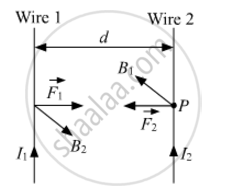# Two Long, Straight, Parallel Conductors Carry Steady Currents, I1 and I2, Separated by a Distance D. If the Currents Are Flowing in the Same Direction, Show How the Magnetic Field Set up in One Produces an Attractive Force on the Other? Obtain the Expression for this Force. Hence, Define One Ampere. - Physics

Two long, straight, parallel conductors carry steady currents, I1 and I2, separated by a distance d. If the currents are flowing in the same direction, show how the magnetic field set up in one produces an attractive force on the other? Obtain the expression for this force. Hence, define one ampere.

#### Solution

Magnetic field induction at some point P on wire 2 due to current Ipassing through wire 1 is given by

B_1=(mu_0 2I_1)/(4pid)As the current-carrying wire 2 lies in magnetic field produced by wire 1, the unit length of wire 2 will experience a force, which is given by

F=B_1I_2xx1=(mu_0)/(4pi) (2I_1I_2)/d

According to Fleming's left-hand rule, the force on wire 2 acts in the plane of paper perpendicular to wire 2, directed towards wire 1. Similarly, wire 1 also experiences the same force towards wire 2.
Thus, both the conducting wires attract each other with the same force F.

Thus, one ampere can be defined as the amount of current flowing through two parallel conductors (in the same direction or opposite directions) placed at a distance of one metre in a free space, and both the wires attract or repel each other with a force of 2×107 Nm1 per metre of their lengths.

Concept: Magnetic Force
Is there an error in this question or solution?
2015-2016 (March) Delhi Set 1

Share